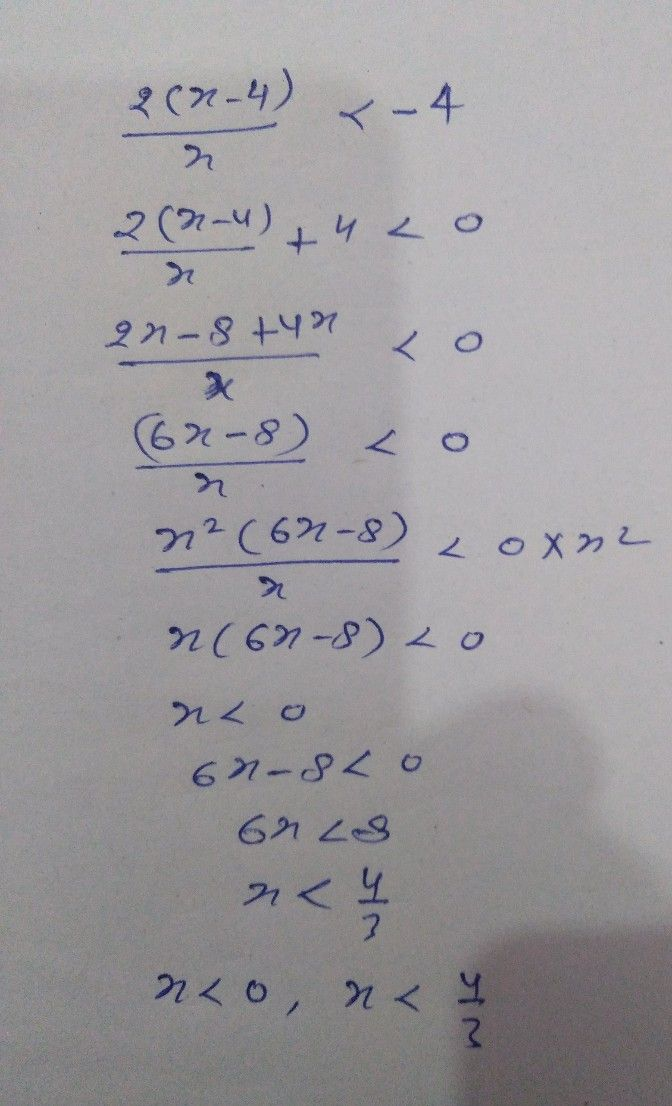Symbol
Problem$3$ $\dfrac {2\left(x-4\right)} {x}<-4$ Rational Inequality $1$ Put the rational inequality in general form. $R\left(x\right)$ $->0$ where $2$ can be replaced by $<,\leq$ and $2$ $2$ Write the inequality into a single rational expression on the left side. $17$
10th-13th grade
Calculus
Search count: 121
SolutionQanda teacher - LokeshStudent
Excuse me sir ahmm, Interval notation or set notation to write the final answer. (0,3/4) in my module